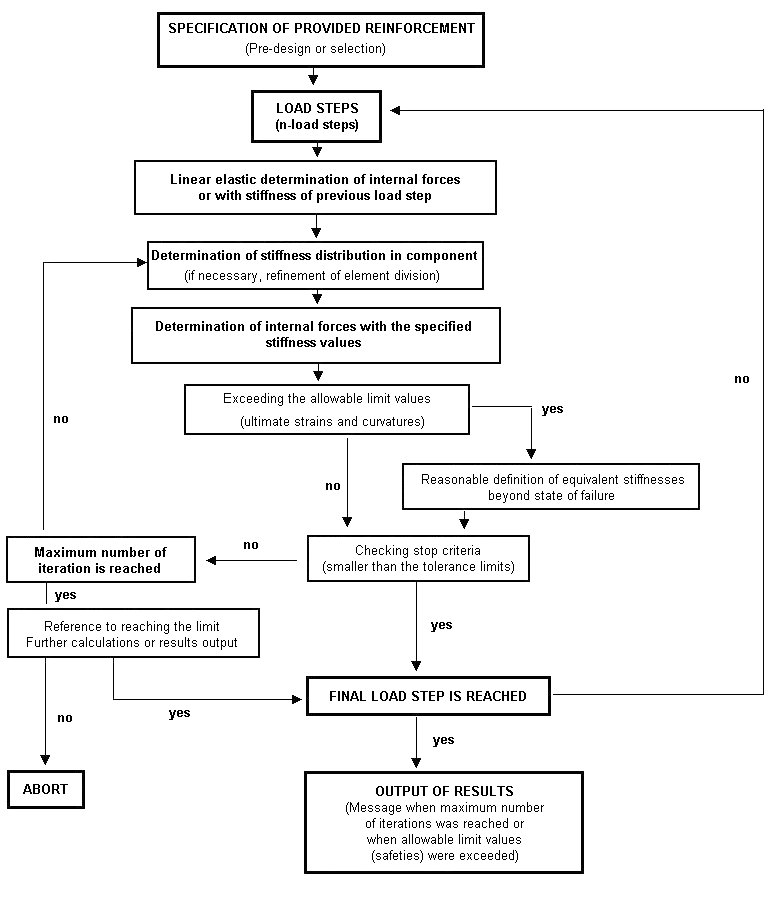# RF-CONCRETE Members – Online Manual Version 5

Online manuals, introductory examples, tutorials, and other documentation.

# 2.4.1 Method

### Method

The principle for the analysis of nonlinear problems is presented by describing an example of uniaxial bending. To determine the nonlinear diagram for deformation of internal forces, the finite element method is used with constant equivalent stiffnesses that are element-by-element. For this reason, the selected division of elements has a significant influence on both the results and the calculation's convergence.

The following flowchart shows the general procedure of a nonlinear calculation.Figure 2.18 General calculation process of nonlinear design

The individual steps are described in the following chapters.